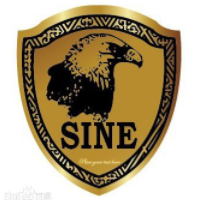# Java 泛型详解

+关注继续查看

#StringClass
public class StringClass {
private String x ;
private String y ;

public String getY() {
return y;
}

public void setY(String y) {
this.y = y;
}

public String getX() {
return x;
}

public void setX(String x) {
this.x = x;
}
}
#IntClass
public class IntClass {
private int x ;
private int y ;

public int getY() {
return y;
}

public void setY(int y) {
this.y = y;
}

public int getX() {
return x;
}

public void setX(int x) {
this.x = x;
}
}

public class ObjClass<T> {

private T x ;
private T y ;

public T getX() {
return x;
}

public void setX(T x) {
this.x = x;
}

public T getY() {
return y;
}

public void setY(T y) {
this.y = y;
}
}

ObjClass<String> stringClass = new ObjClass<String>();
stringClass.setX("haha");

ObjClass<Integer> intClass = new ObjClass<Integer>();
intClass.setX(100);

Log.d("yyy", "stringClass:" + stringClass.getX() + ",intClass:" + intClass.getX());

1.首先需要定义泛型：ObjClass

ObjClass ，即在类名后面加一个尖括号，括号里是一个大写字母。这里写的是T，其实这个字母可以是任何大写字母，无论使用哪个字母，意义都是相同的。如果你想学习Java可以来这个群，首先是二二零，中间是一四二，最后是九零六，里面有大量的学习资料可以下载

2.在类中使用泛型

private T x ;
private T y ;

public T getX() {
return x;
}

public void setX(T x) {
this.x = x;
}

public T getY() {
return y;
}

public void setY(T y) {
this.y = y;
}
3.使用泛型类

ObjClass<String> stringClass = new ObjClass<String>();
stringClass.setX("haha");

ObjClass<Integer> intClass = new ObjClass<Integer>();
intClass.setX(100);

ObjClass<String> stringClass = new ObjClass<String>();

1.多泛型变量定义

public class ObjClass<T,U>

public class ObjClass<T,U> {

private T x ;
private U y ;

public T getX() {
return x;
}

public void setX(T x) {
this.x = x;
}

public U getY() {
return y;
}

public void setY(U y) {
this.y = y;
}
}
ObjClass<String,Integer> stringClass = new ObjClass<String,Integer>();
stringClass.setX("haha");
stringClass.setY(100);

2.泛型的字母规范

E — Element，常用在java Collection里，如：  List<E>,Iterator<E>,Set<E>
K,V — Key，Value，代表Map的键值对
N — Number，数字
T — Type，类型，如String，Integer等等

interface MsgClass<T> {
public T getMsg() ;
public void setMsg(T x);
}

public class Message<T,U> implements MsgClass<T>{

private T msg;
@Override
public T getMsg() {
return msg;
}

@Override
public void setMsg(T msg) {
this.msg = msg;
}
}

public class Message<T,U> implements MsgClass<T>{
private U name;
private T msg;
@Override
public T getMsg() {
return msg;
}

@Override
public void setMsg(T msg) {
this.msg = msg;
}

public U getName() {
return name;
}

public void setName(U name) {
this.name = name;
}
}

public class ObjClass {
//静态函数
public static <T> void StaticMethod(T a) {

}

//普通函数
public <T> void OrgnicMethod(T a) {

}

}

//静态方法
//常规方法
ObjClass objClass = new ObjClass();
objClass.OrgnicMethod(new Integer(111));//使用方法一
objClass.<Integer>OrgnicMethod(new Integer(111));//使用方法二

public static <T> List<T> parseArray(String response,Class<T> object){
List<T> modelList = JSON.parseArray(response, object);
return modelList;
}

//定义
public static <T> T[] fun1(T...msg){  // 接收可变参数
return msg ;            // 返回泛型数组
}
//使用
public static void main(String args[]){
Integer i[] = fun1(8,9,8,44) ;
Integer[] result = fun1(i) ;
}

class Info<T>{
private T var ;        // 定义泛型变量
public void setVar(T var){
this.var = var ;
}
public T getVar(){
return this.var ;
}
public String toString(){
return this.var.toString() ;
}
};

public class demo1 {
public static void main(String args[]) {
// 使用String为泛型类型
Info<String> i = new Info<String>();
i.setVar("ABCD");
//把String泛型类型的i对象传递给Object泛型类型的temp。
fun(i);
}

// 接收Object泛型类型的Info对象
public static void fun(Info<Object> temp) {
System.out.println("内容：" + temp);
}
}

Exception in thread "main" java.lang.Error: Unresolved compilation problem:
The method fun(Info<Object>) in the type demo1 is not applicable for the arguments (Info<String>)

class Info<T>{
private T var ;
public void setVar(T var){
this.var = var ;
}
public T getVar(){
return this.var ;
}
public String toString(){
return this.var.toString() ;
}
};
public class GenericsDemo{
public static void main(String args[]){
Info<String> i = new Info<String>() ;
i.setVar("ABCD") ;
fun(i) ;
}
public static void fun(Info<?> temp){
System.out.println("内容：" + temp) ;
}
};

class Info<T>{
private T var ;
public void setVar(T var){
this.var = var ;
}
public T getVar(){
return this.var ;
}
public String toString(){
return this.var.toString() ;
}
};
public class demo1{
public static void main(String args[]){
Info<?> i = new Info<String>() ;
i.setVar("ABCD") ;
}
};

Exception in thread "main" java.lang.Error: Unresolved compilation problem:
The method setVar(capture#1-of ?) in the type Info<capture#1-of ?> is not applicable for the arguments (String)

class Info<T>{
private T var ;
public void setVar(T var){
this.var = var ;
}
public T getVar(){
return this.var ;
}
public String toString(){
return this.var.toString() ;
}
};
public class GenericsDemo{
public static void main(String args[]){
Info<Integer> i1 = new Info<Integer>() ;
Info<Float> i2 = new Info<Float>() ;
i1.setVar(30) ;
i2.setVar(30.1f) ;
fun(i1) ;
fun(i2) ;
}
public static void fun(Info<? extends Number> temp){    // 只能接收Number及其Number的子类
System.out.print(temp + "、") ;
}
};

class Info<T extends Number>{    // 此处泛型只能是数字类型
private T var ;
public void setVar(T var){
this.var = var ;
}
public T getVar(){
return this.var ;
}
public String toString(){
return this.var.toString() ;
}
};
public class demo1{
public static void main(String args[]){
Info<Integer> i1 = new Info<Integer>() ;        // 声明Integer的泛型对象
}
};

<? super XXX> 表示填充为任意XXX的父类

class Info<T>{
private T var ;
public void setVar(T var){
this.var = var ;
}
public T getVar(){
return this.var ;
}
public String toString(){
return this.var.toString() ;
}
};
public class GenericsDemo21{
public static void main(String args[]){
Info<String> i1 = new Info<String>() ;        //
Info<Object> i2 = new Info<Object>() ;        //
i1.setVar("hello") ;
i2.setVar(new Object()) ;
fun(i1) ;
fun(i2) ;
}
public static void fun(Info<? super String> temp){    // 只能接收String或Object类型的泛型，String类的父类只有Object类
System.out.print(temp + "、") ;
}
};
Object类和String类都是String的父类，所有运行成功，但是如果此时用Integer则会出错，因为integer并不是String父类。

class CEO extends Manager {
}

class Manager extends Employee {
}

class Employee {
}

List<? super Manager> list;
list = new ArrayList<Employee>();
//存

List<Employee> list = new ArrayList<Employee>();

List<? super Manager> list;
list = new ArrayList<Employee>();
//存

2）泛型的上限：？extends 类型（能取不能存）。

3）泛型的下限：？super 类型? super 通配符（能存不能取）。22599 03126 09420 06925 04496 0Xshell使用SSH远程登录阿里云ECS服务器CentOS7
10188 0windows server 2008阿里云ECS服务器安全设置

5460 03830 046886 03229 0

web前端新手群291851189
217

0

《SaaS模式云原生数据仓库应用场景实践》

《看见新力量：二》电子书